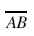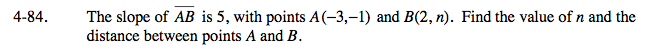### Home > A2C > Chapter 4 > Lesson 4.2.1 > Problem4-84

4-84.

The slope ofis 5, with points A(−3, −1) and B(2, n). Find the value of n and the distance between points A and B. Homework Help ✎Use the slope and the given point to write an equation.

Substitute into your equation to find n.

Now that you know the coordinates of both points, find the distance between them.

The distance between the points is

$5\sqrt{26}$